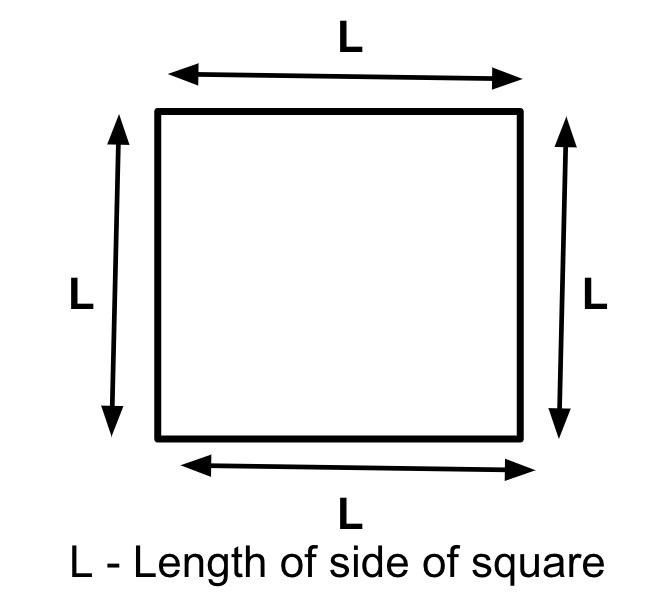# How to Find Area of Square in Golang?

In this tutorial, we are going to see the Golang program to find the Area of a Square. The area is the total space covered by any closed figure.## Formula

Area of Square - (length of a side) * (length of a side)
s - length of a side of a Square


For example, the length of a side of a Square is 10 cm so the Area of a Square is −

Area = 10 * 10
= 100 cm^2


## Finding the Area of a Square within the function

### Algorithm

Step 1 − Declaring the variables for the length of a side and the area of the float64 data type.

Step 2 − Initialize the variable.

Step 3 − Find the Area using the above formula within the function.

Step 4 − Printing the result.

Time Complexity:
O(1)

Space Complexity:
O(1)


## Example 1

In this example, we are going to find the Area of a Square within the function.

package main
// fmt package provides the function to print anything
import (
"fmt"
)
func main() {
// declaring the floating variables using the var keyword for
// storing the length of the side of the Square also a variable area
// to store Area
var lengthOfSide, Area float64
fmt.Println("Program to find the Area of a Square.")

// initializing the length of the side of a Square
lengthOfSide = 10

// finding the Area of a Square
Area = (lengthOfSide * lengthOfSide)

// printing the result
fmt.Println("The Area of a Square whose length of the side is", lengthOfSide, "is", Area, "cm * cm.")
fmt.Println("(Finding the Area of a Square within the function)")
}


## Output

Program to find the Area of a Square.
The Area of a Square whose length of the side is 10 is 100 cm * cm.
(Finding the Area of a Square within the function)


## Description of code

• var lengthOfSide, Area float64 − In this line, we are declaring the length of a side and the Area that we are going to use later. As the length of a side or Area can be in decimal, we have used the float data type.

• Area = (lengthOfSide * lengthOfSide) − In this line of code, we are applying the formula and finding the Area.

• fmt.Println("The Area of a Square whose length of side is", lengthOfSide, "is", Area, "cm * cm.")

− Printing the Area of a Square.

## Finding the Area of a Square in the separate function

### Algorithm

Step 1 − Declaring the variables for the length of a side and the area of the float64 data type.

Step 2 − Initialize the variable.

Step 3 − Calling the function with the length of a side as a parameter, and storing the Area the function is returning.

Step 4 − Printing the result.

## Example 2

In this example, we are going to find the Area of a Square by defining the separate function to find the Area.

package main
// fmt package provides the function to print anything
import (
"fmt"
)
func areaOfSquare(lengthOfSide float64) float64 {
// returning the area by applying the formula
return (lengthOfSide * lengthOfSide)
}
func main() {
// declaring the floating variables using the var keyword for
// storing the length of the side of the Square also a variable area
// to store Area
var lengthOfSide, Area float64
fmt.Println("Program to find the Area of a Square.")

// initializing the length of the side of a Square
lengthOfSide = 10

// finding the Area of a Square by calling areaOfSquare() function
Area = areaOfSquare(lengthOfSide)

// printing the result
fmt.Println("The Area of a Square whose length of a side is", lengthOfSide, "is", Area, "cm * cm.")
fmt.Println("(Finding the Area of a Square in the separate function)")
}


## Output

Program to find the Area of a Square.
The Area of a Square whose length of a side is 10 is 100 cm * cm.
(Finding the Area of a Square in the separate function)


## Description of code

• var lengthOfSide, Area float64 − In this line, we are declaring the length of a side and the Area that we are going to use later. As the length of a side, or Area can be in decimal so we have used the float data type.

• Area = areaOfSquare(lengthOfSide) − In this line of code, we call the function finding the Area of a Square.

• fmt.Println("The Area of a Square whose length of side is", lengthOfSide, "is", Area, "cm * cm.")

Printing the Area of a Square.

## Conclusion

These are the two ways to find the Area of a Square in Golang. The second way is much better in terms of modularity and code reusability as we can call that function anywhere in the project. To learn more about go you can explore these tutorials.